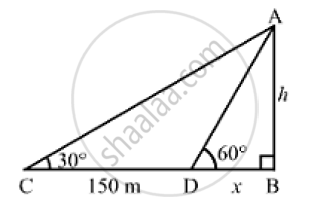# The Angle of Elevation of the Top of a Tower from Ta Point on the Same Level as the Foot of the Tower is 30° ,Show that the Height of the Tower is 129.9 Metres. - Mathematics

The angle of elevation of the top of a tower from ta point on the same level as the foot of the tower is 30° . On advancing 150 m towards foot of the tower, the angle of elevation becomes 60° Show that the height of the tower is 129.9 metres.

#### Solution

Let AB be the tower
We have:
CD =150m,∠ACB = 30° and ∠ADB = 60°
Let:
AB = hm and BD = xmIn the right ΔABD,we have:

(AB)/(AD) = tan 60° = sqrt(3)

⇒h/x = sqrt(3)

⇒ x = h/sqrt(3)

Now, in the right  ΔACB,we have:

(AB)/(AC) = tan 30° = 1/ sqrt(3)

⇒ h/(x +150) = 1/ sqrt(3)

sqrt(3) h =x +150

On putting x= h/ sqrt(3)  in the above equation, we get:

sqrt(3)h = h/ sqrt(3) + 150

⇒ 3h = h + 150 sqrt(3)

⇒ 2h = 150sqrt(3)

⇒ h = (150 sqrt(3)) /2 =75 sqrt(3) = 75 xx1.732 = 129.9m`

Hence, the height of the tower is 129.9 m

Concept: Heights and Distances
Is there an error in this question or solution?
Chapter 14: Height and Distance - Exercises

#### APPEARS IN

RS Aggarwal Secondary School Class 10 Maths
Chapter 14 Height and Distance
Exercises | Q 26

Share